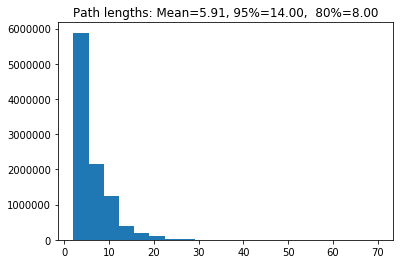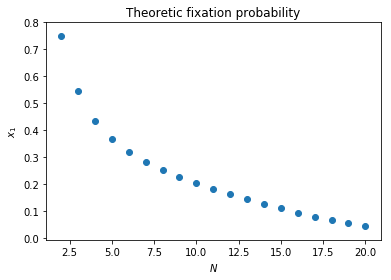In :
%matplotlib inline

import collections

import matplotlib.pyplot as plt
import numpy as np

In :
def roll_n_sided_dice(n=6):
"""
Roll a dice with n sides.
"""
return np.random.randint(1, n + 1)

class MoranProcess:
"""
A class for a moran process with a population of
size N=3 using the standard Hawk-Dove Game:

A =
[0, 3]
[1, 2]

Note that this is a simulation corresponding to an
in class activity where students roll dice.
"""
def __init__(self, number_of_hawks=1, seed=None):

if seed is not None:
np.random.seed(seed)

self.number_of_hawks = number_of_hawks
self.number_of_doves = 3 - number_of_hawks

self.dice_and_values_for_hawk_birth = {1: (6, {1, 2, 3}), 2: (4, {1, 2, 3})}
self.dice_and_values_for_hawk_death = {1: (6, {1, 2}), 2: (6, {1, 2, 3, 4})}

self.history = [(self.number_of_hawks, self.number_of_doves)]

def step(self):
"""
Select a hawk or a dove for birth.
Select a hawk or a dove for death.

Update history and states.
"""
birth_dice, birth_values = self.dice_and_values_for_hawk_birth[self.number_of_hawks]
death_dice, death_values = self.dice_and_values_for_hawk_death[self.number_of_hawks]

select_hawk_for_birth = self.roll_dice_for_selection(dice=birth_dice, values=birth_values)
select_hawk_for_death = self.roll_dice_for_selection(dice=death_dice, values=death_values)

if select_hawk_for_birth:
self.number_of_hawks += 1
else:
self.number_of_doves += 1

if select_hawk_for_death:
self.number_of_hawks -= 1
else:
self.number_of_doves -= 1

self.history.append((self.number_of_hawks, self.number_of_doves))

def roll_dice_for_selection(self, dice, values):
"""
Given a dice and values return if the random roll is in the values.
"""
return roll_n_sided_dice(n=dice) in values

def simulate(self):
"""
Run the entire simulation: repeatedly step through
until the number of hawks is either 0 or 3.
"""
while self.number_of_hawks in [1, 2]:
self.step()
return self.number_of_hawks

def __len__(self):
return len(self.history)

In :
repetitions = 10 ** 7
end_states = []
path_lengths = []
for seed in range(repetitions):
mp = MoranProcess(seed=seed)
end_states.append(mp.simulate())
path_lengths.append(len(mp))

In :
counts = collections.Counter(end_states)
counts / repetitions

Out:
0.5452601
In :
plt.hist(path_lengths, bins=20)
plt.title("Path lengths: Mean={:0.2f}, 95%={:0.2f},  80%={:0.2f}".format(np.mean(path_lengths),
np.percentile(path_lengths, 95),
np.percentile(path_lengths, 80)));Note that this is not how we would do this in practice:

In :
P = np.array([[1, 0, 0, 0],
[1 / 6, 1 / 2, 1 / 3, 0],
[0, 1 / 6, 7 / 12, 1 / 4],
[0, 0, 0, 1]])
np.round(np.linalg.matrix_power(P, 100), 4)

Out:
array([ 0.4545,  0.    ,  0.    ,  0.5455])
In :
def theoretic_fixation(N, game, i=1):
"""
Calculate x_i
"""
f_ones = np.array([(game[0, 0] * (i - 1) + game[0, 1] * (N - i)) / (N - 1) for i in range(1, N)])
f_twos = np.array([(game[1, 0] * i + game[1, 1] * (N - i - 1)) / (N - 1) for i in range(1, N)])
gammas = f_twos / f_ones
return (1 + np.sum(np.cumprod(gammas[:i-1]))) / (1 + np.sum(np.cumprod(gammas)))

In :
theoretic_fixation(N=3, game=np.array([[0, 3], [1, 2]]), i=1)

Out:
0.54545454545454553
In :
Ns = range(2, 20 + 1)
fixations = [theoretic_fixation(N=n, game=np.array([[0, 3], [1, 2]]), i=1)
for n in Ns]
plt.scatter(Ns, fixations)
plt.xlabel("$N$")
plt.ylabel("$x_1$")
plt.title("Theoretic fixation probability");